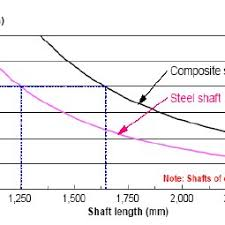## How to Calculate and Solve for Shaft Length | Ball Mill SizingThe image above represents shaft length.

To compute for shaft length, four essential parameters are needed and these parameters are Applied Torque (T), Length of Shaft (L), Modulus of Rigidity (G) and Diameter of Shaft (D).

The formula for calculating shaft length:

θ = T x L x 32/G x π x D4

Where:

θ = Shaft Length
T = Applied Torque
L = Length of Shaft
G = Modulus of Rigidity
D = Diameter of Shaft

Let’s solve an example;
Find the shaft length when the applied torque is 8, the length of shaft is 12, the modulus of rigidity is 4 and the diameter of shaft is 10.

This implies that;

T = Applied Torque = 8
L = Length of Shaft = 12
G = Modulus of Rigidity = 4
D = Diameter of Shaft = 10

θ = T x L x 32/G x π x D4
θ = (8) x (12) x 32/(4) x π x (10)4
θ = 3072/(4) x π x (10000)
θ = 3072/125663.7
θ = 0.024

Therefore, the shaft length is 0.024 m.

Calculating the Applied Torque when the Shaft Length, the Length of Shaft, the Modulus of Rigidity and the Diameter of Shaft is Given.

T = θ x G x π x D4 / L x 32

Where;

T = Applied Torque
θ = Shaft Length
L = Length of Shaft
G = Modulus of Rigidity
D = Diameter of Shaft

Let’s solve an example;
Find the applied torque when the shaft length is 10, the length of shaft is 5, the modulus of rigidity is 4 and the diameter of shaft is 2.

This implies that;

θ = Shaft Length = 10
L = Length of Shaft = 5
G = Modulus of Rigidity = 4
D = Diameter of Shaft = 2

T = θ x G x π x D4 / L x 32
T = 10 x 4 x π x 24 / 5 x 32
T = 40 x π x 16 / 160
T = 2010.6 / 160
T = 12.56

Therefore, the applied torque is 12.56 m.